# Personal identification based on digital retinal images

Introduction

Recognition of a person on the basis of retinal image is biometric procedure which uses the blood vessels pattern in the retina for recognition. Although it is not as popular as other identification procedures such as finger print, voice recognition, DNA analysis or iris or face recognition, retinal images recognition has some advantages over other techniques.

For example, when compared to finger print recognition it is more robust and precise. Fingerprint recognition is more sensitive to different finger position, scars and filths. When compared to voice recognition there is no problem of background noise and retina is less sensitive to changes due to illnesses or state of person’s health and mood. DNA analysis has huge disadvantage over most of other techniques; long processing time.

Drawbacks of retinal image recognition are somewhat uncomfortable process of taking picture, expensive equipment and potential changes of retina due to illnesses and astigmatism. This recognition technique is still not widely used. Most often it is used for gaining access to complexes with very high level of security. It is used by some of governmental agencies in USA, such as FBI, CIA and NASA.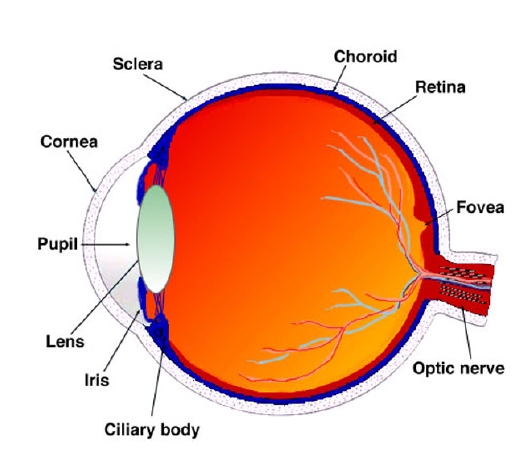Theoretical background

Figure above shows an eye with the marked most important parts. The figure shows that the retina is the inner membrane and is placed in the back of the eye. When the eye is focused, the light is projected on the retina. Due to the complex structure of capillaries that supply the retina with blood, the retina of every person is unique. The network of capillaries in the retina is not genetically determined so that even in identical twins this network is not similar. Although blood vessels in the retina can be changed as a result of diabetes, glaucoma or retinal disorders, retina remains unchanged from birth to death. For these reasons, the identification by the image of the retina is one of the most accurate biometric identification methods. The inaccuracy of this method is one of 10 million.

Image of the retina is obtained by a scanner which emits an infrared beam of low energy in the eye of the person looking into the eyepiece scanner. Beam moves over the retina by a standardized way. The capillaries in the retina absorb more light than tissue that surrounds them so that reflection during scanning varies. After that, the images are digitized and processed.

The algorithm that is used is divided into three steps. In the first step, the method of amplitude segmentation determines the center of optical disk. In the second step, the modified filter produces binary image in which vessels are expressed. In third step, the two binary images of the retinas are aligned by the centers obtained in the second step and then their difference is determined and, based on result it is decided whether the two images are from the same person.

Algorithm

The first task of the embodiment consists of finding of position of an optical disc. Optical disk area is the brightest part of the image and therefore its location can be determined by the amplitude image segmentation. This position is required later for the alignment of images in order to make their comparison.

First we need to blur the image by convolution with averaging filter. Then the extraction of middle part of the picture is done. This step is required because of the possible appearance of bright parts on the edges of the image that could affect the accuracy of the solution. It is extracted by multiplying the elements of the original image with a mask consisting of the units in the center and a zero at the edges. Since the optical disc is on all images located near the middle of the picture, removal of the peripheral areas does not lead to the loss of the accuracy of detection.

Following the removal of unnecessary parts, the brightest part of the picture has to be found. The first step in this procedure is the preparation of the histogram and the percentages of the light part. It was experimentally obtained that for most cases the best results are obtained when it is assumed that the proportion of bright areas is approximately 1.5% of the total area of the histogram. Then binary mask is calculated using the boundary calculated from the histogram. The last step is to calculate the coordinates of boundaries of optical disc from these masks. The result of detection of the optical disk is shown in figure below.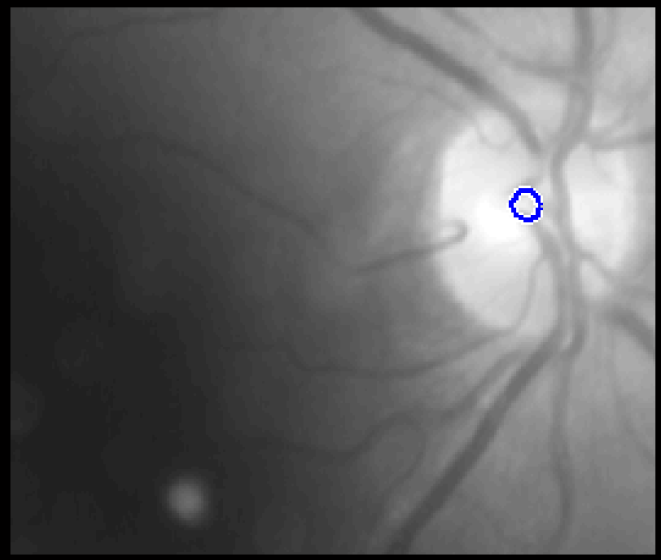The second task is the detection of vessels using the matched filter. In contrast to the detection of the edges, the matched filter takes into account the shape of the object to be detected and gives results with much less discontinuity. When designing matched filter four characteristics of blood vessels in retinal images were observed:

1. Blood vessel have a small curvature and can be approximated with array of linear segments
2. Since they reflect light less than other parts of the retina, they look darker than the background. The vessels almost never have sharp edges. Although the intensities of the various vessels vary, they can be approximated by a Gaussian curve.
3. Although the width of the vessels decreases as the vessel moves away from the optical disc, such a change is gradual.
4. It can be assumed that the vessels are symmetrical in length.

Matched filter should have the form of an object that we want to detect. Taking into account the above listed characteristics of vessels, matched filter had a form: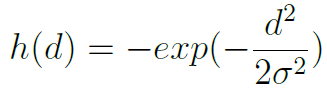where d is the width of blood vessels, and σ determines the width of Gaussian curve.

In two-dimensional space it is necessary to consider that the vessels may occur at any angle and that the filter has to be rotated to all possible angles (0-180). In theory Gaussian curve is of infinite length so here it is determined that will be cut to ±3σ. Length of vessel segments that we want to detect is indicated by L. Thus, the size of the mask that defines a custom filter is L*6σ. The formula for calculating the coefficients of the masks is: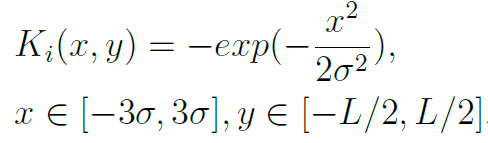Following already said, mask has the following form: L same lines where the line is limited by Gaussian function. In our case we selected L = 9 and σ=2. For the default parameters mask is shown in the picture below.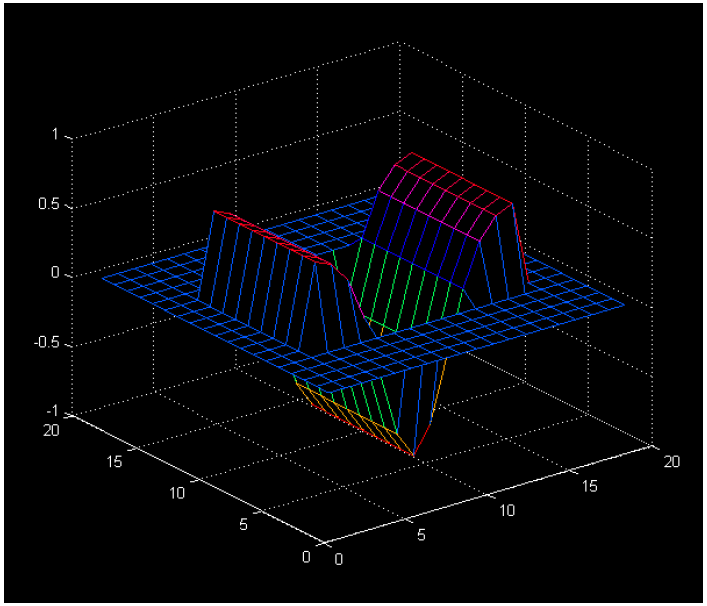After generating the mask it needs to set its mean value to 0. This is done so that the mean value is calculated by the formula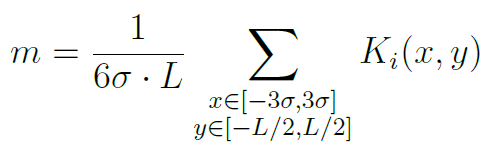and thereafter from each coefficient subtracting this mean value. Then the mask is normalized by dividing with the maximum coefficient in the mask.

So that the coefficients of the mask would not be lost in the rotation, the mask is extended on each side with zeros. This allows usage of a mask of the same size for every angle of filtration. First, the mask is rotated for a certain angle and then the convolution is performed. The binary image is obtained by choosing the threshold value from convolution result. The threshold is determined automatically so that 4% points with maximum values after filtration are declared as vessels. Increment step of angle in this case was 10 degrees. After rotation for all angles, the images are combined using the OR operation into a single image. At the end of the binary image, objects that are smaller than 125 pixels are removed, in order to remove slightly detected blood vessel and other unimportant objects. The result of detection of vessels with mentioned algorithm is shown in figure below.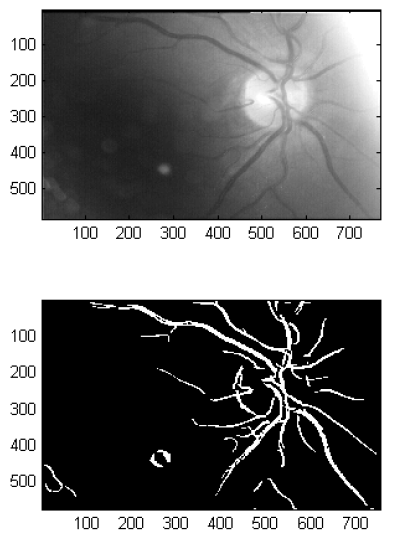Decision whether to two different images of the retina belong to the same person shall be based on the difference of previously calculated binary image. The problem in the process of subtraction is fact that the images are often translated and rotated for a smaller angle. To solve this problem it is necessay first to set a reference point in the pictures. The selected reference point is the center of the optical disk obtained by amplitude segmentation and it serves to align the image in the process of subtraction. The difference image is calculated by subtracting two binary images. The process of subtraction begins by subsampling the images for a factor 2 in order to reduce the calculation time. After that it is necessary to expand the image and overlap them. As seen in figure below, overlapping the original images usually gives some major deviations. To achieve the best possible overlap, one image is taken as a reference, and the other is translated to positive and negative horizontal and vertical direction by 25 pixels with the steps of 1 pixel and rotated from -5 to +5 degrees in steps of 0.5 degrees. After each transformation the difference of two images is computed and result is stored in order to find the smallest value.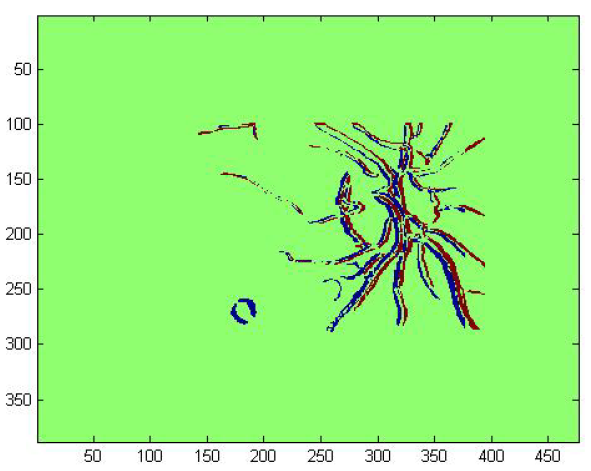After the series of rotations and shifts the smallest difference is obtained, and now it is needed to decide on whether the images of the retina belong to the same person. This process begins with the rotation and translation of one image with the obtained parameters for which the smallest difference was calculated. After that one binary image is multiplied by a factor of two, and the second binary image is subtracted from it. In the newly created image the pixels with value 1 represent the overlapping vessels. The measure for the final decision is a percentage of overlapping pixels in the new image. Example of two image differences is visible and calculation of overlapping is shown in figures below.

.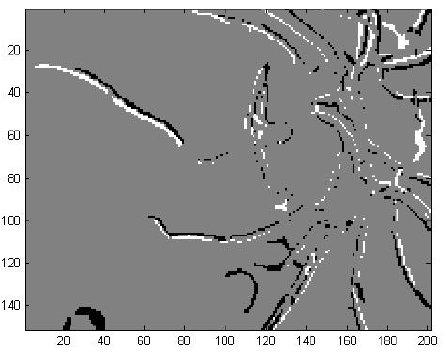Results

For most images algorithm gives good results. For pictures of the same person retina which are slightly different algorithm gives the percentage of pixels aligned above 90%. For pictures of the same person retina which are very different, for example, different contrast and brightness or a large difference between the centers of the optic nerves, percentage of alignment is between 60% and 70%. For images of different people’s retinas, aligned percentage is between 20% and 30%.

The inability to recognize can occur when on one of the image optical disc is not well recognized. An example of this case is shown in figure below. In figure optical drive is not properly recognized and is far from the true origins of the nerve. Erroneous determination of the optical disk comes mostly with images that are brighter than the average brightness of all images of the retina in the database. Then the selected value of 1.5% for determining the optical disc is not sufficient and false detection occurs.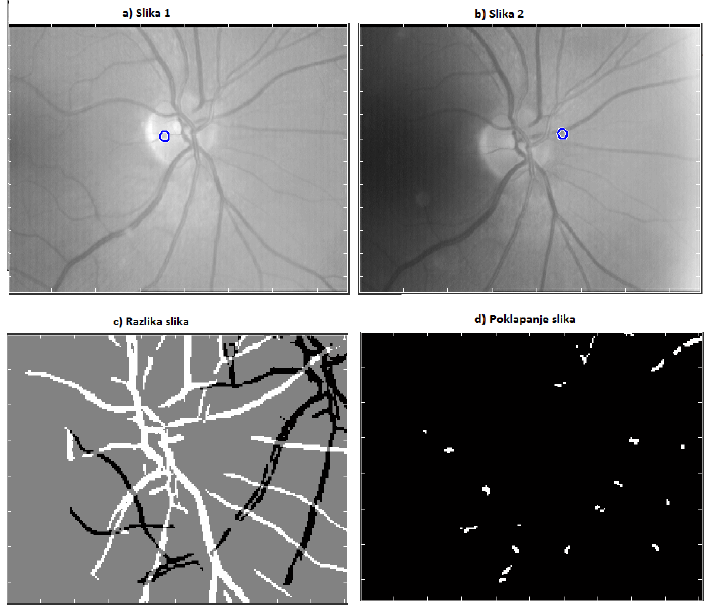Conclusion

Recognition of a person based on the image of the retina is a technique that is not very extensive, but has a number of advantages over the procedures for identification which are currently widely used. Retina is unique to each person and is very resistant to change. Moreover, a network of vessels in the retina is very complex. These properties make the image of the retina interesting for an application of identifying persons.

Given that the two images of the same retina are not completely identical, and that is possible to have a small translation or rotation when capturing an image, it is necessary to determine an element in the image as a reference. The ideal showed an optical disc. The area in most cases is the brightest area in the image. In addition, in this part, the network of vessels is the densest and most complex, so it is enough to make comparisons between image area located in its immediate vicinity.

In order to prevent the influence of the brightness of image on the results it is necessary to detect only parts relevant for comparison; that is network of vessels. Detection of vessels was performed using matched filter because it takes into account the shape of the object that we want to detect and gives results with much less discontinuity. Once we have extracted all the necessary data, the comparison of two images from databases followed. When comparing, one image is taken as a reference, while the other is translated and rotated. When the best fit is found the percentage of overlapping pixels from the original image is calculated. On the basis of this number it is concluded whether the images belong to the same person.

Acknowledgement

This project was a project on a Digital Image Processing and Analysis course and was done in a group of four students: Antonio Benc, Ante Gojsalić, Lucija Jurić and me. The final report can be seen here (in Croatian unfortunately):  Project_report and the presentation (in English) here:   Project_presentation

Literature

 C. Marino, M. G. Penedo, M. Penas, M. J. Carreira F. Gonzales, “Personal authentication using digital retinal images”, Springer-Verlag London Limited, January 2006

 S. Chaudhuri, S. Chatterjee, N. Katz M. Nelson, M. Goldbaum, “Detection of Blood Vessels in Retinal Images Using Two-Dimensonal Matched Filters”, IEEE Transactions on Medical Imaging, vol. 8, no. 3, September in 1989.